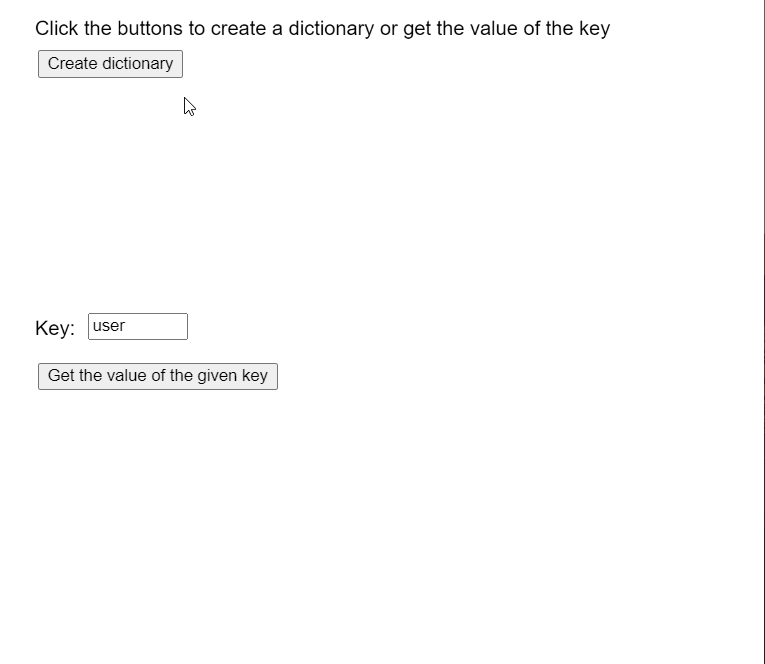# p5.js TypedDict get() Method

• Last Updated : 25 Nov, 2020

The get() method of p5.TypedDict in p5.js is used to return the value at the given key of the dictionary. The method returns undefined if the key does not exist in the dictionary. A key-value pair is a set of two values that are mapped to each other. These values can be accessed by querying this dictionary using the key portion of the pair. A typed dictionary can store multiple key-value pairs that can be accessed using the methods of the dictionary.

Syntax:

Hey geek! The constant emerging technologies in the world of web development always keeps the excitement for this subject through the roof. But before you tackle the big projects, we suggest you start by learning the basics. Kickstart your web development journey by learning JS concepts with our JavaScript Course. Now at it's lowest price ever!

```get( key )
```

Parameters: This method accepts a single parameter as shown above and discussed below:

• key: This is a number or string that denotes the key that has to be added to the dictionary.

Return Value: This method returns a number or string that denotes the value stored at the given key.

The example below illustrates the get() method in p5.js:

Example:

## Javascript

 `let y = 0;`` ` `function` `setup() {``  ``createCanvas(550, 500);``  ``textSize(16);`` ` `  ``text(``"Click the buttons to create a "` `+``       ``"dictionary or get the value of the key"``,``       ``20, 20);`` ` `  ``text(``"Key:"``, 20, 260);`` ` `  ``key_input = createInput(``'user'``);``  ``key_input.position(70, 250);``  ``key_input.size(80);`` ` `  ``setBtn = ``    ``createButton(``"Create dictionary"``);``  ``setBtn.position(30, 40);``  ``setBtn.mouseClicked(createNewDict);`` ` `  ``getBtn = ``    ``createButton(``"Get the value of the given key"``);``  ``getBtn.position(30, 290);``  ``getBtn.mouseClicked(getVal);``}`` ` `function` `createNewDict() {``  ``clear();`` ` `  ``// Create an object with random values``  ``let obj = {};``  ``for` `(let i = 0; i < 5; i++) {``    ``let rk = ceil(Math.random() * 100);``    ``let rn = floor(Math.random() * 100);`` ` `    ``let rkey = ``"user"` `+ rk;``    ``let rval = ``"data"` `+ rn;``    ``obj[rkey] = rval;`` ` `    ``text(``"Key: "` `+ rkey + ``"  Value: "` `+``         ``rval, 40, 120 + 20 * i);``  ``}`` ` `  ``// Create a string dict using the above values``  ``numDict = createStringDict(obj);`` ` `  ``text(``"New Dictionary created with values"``,``       ``20, 80);`` ` `  ``text(``"Click the buttons to create a "` `+``       ``"dictionary or get the value of the key"``,``       ``20, 20);`` ` `  ``text(``"Key:"``, 20, 260);``}`` ` `function` `getVal() {`` ` `  ``// Get the key to be retrieved``  ``let keyToCheck = key_input.value();`` ` `  ``// Get the value of the key from the dictionary``  ``let keyVal = numDict.get(keyToCheck);`` ` `  ``text(``"The value at key: "` `+ keyToCheck +``       ``" is: "` `+ keyVal, 20, 340 + y * 20);`` ` `  ``y++;`` ` `  ``text(``"Click the buttons to create a "` `+``       ``"dictionary or get the value of the key"``,``       ``20, 20);``}`

Output:Online editor: https://editor.p5js.org/
Environment Setup: https://www.geeksforgeeks.org/p5-js-soundfile-object-installation-and-methods/
Reference: https://p5js.org/reference/#/p5.TypedDict/get

My Personal Notes arrow_drop_up